# Exploring MNIST Dataset using PyTorch to Train an MLP

From the visual search for improved product discoverability to face recognition on social networks- image classification is fueling a visual revolution online and has taken the world by storm. Image classification, a subfield of computer vision helps in processing and classifying objects based on trained algorithms. Image Classification had its Eureka moment back in 2012 when Alexnet won the ImageNet challenge and since then there has been an exponential growth in the field. While we humans take our ability to easily classify objects surrounding us because our brains have been trained unconsciously with the same set of images, the problem is not that easy after all. Several factors like view-point variation, size variation, occlusion(blending of objects with other objects in the image), differences in the direction and source of light make it difficult for machines to classify images correctly. Nonetheless, it is an exciting and growing field and there can't be a better way to learn the basics of image classification than to classify images in the MNIST dataset.

## What is the MNIST dataset?

Before we go any further let's see what is MNIST dataset.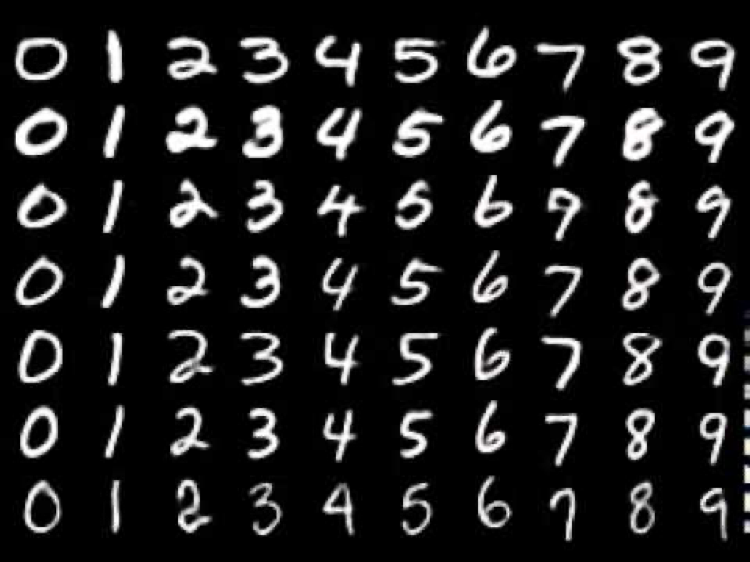MNIST stands for Modified National Institute of Standards and Technology and is a database of 60,000 small square 28x28 pixel grayscale images. MNIST handwritten digits dataset is the most used for learning Image Recognition. It is labeled in the sense that each image of a handwritten digit has the corresponding numeral value attached to it. This helps our Algorithm/Neural Network to learn which image stands for which number (0-9) and to learn hidden patterns in human writing.

Learn to Classify Handwritten Digits Using MNIST Dataset

## Types of MNIST Dataset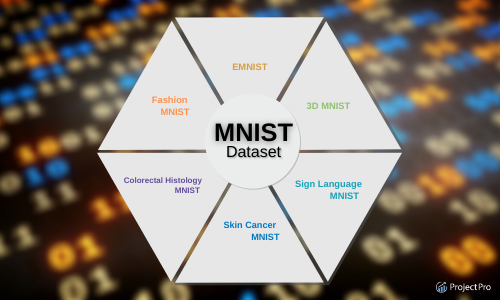While the handwritten MNIST is the most popular one, there are 6 different extended variations of MNIST:

1) Fashion MNIST: This dataset from Zalando Research contains images of 10 classes consisting of clothing apparel and accessories like ankle boots, bags, coats, dresses, pullovers, sandals, shirts, sneakers, etc. instead of handwritten digits. The images are grayscale just like the original MNIST.

2) 3D MNIST: While the original MNIST has 28X28 grayscale (one channel) images, 3D MNIST has images with 3 channels (vis. Red, Green, Blue) like any other color-image out there. It provides a good way to start with 3D Computer Vision Problems.

3) EMNIST: EMNIST is a set of handwritten letters contrary to MNIST which only has handwritten digits. The structure is pretty much the same as MNIST containing grayscale 28X28 images.

4) Sign Language MNIST:  It is like EMNIST, in the sense that it has images of sign language interpretations of the English alphabets(A-Z). It poses a little more challenging problem of hand gesture recognition and therefore has more useful real-world applications.

5) Colorectal Histology MNIST: The dataset serves a much more interesting MNIST problem for biologists by focusing on histology tiles from patients with colorectal cancer - affecting colon or rectum in the human body. In particular, the data has 8 different classes of cancerous tissue.

6) Skin Cancer MNIST: It is a medical dataset containing images of skin lesions/cancers along with their corresponding labels. This dataset was made for the 2018 Skin Lesion Detection Challenge. It can be used as a primary dataset for anyone trying to tackle a medical classification problem using deep learning.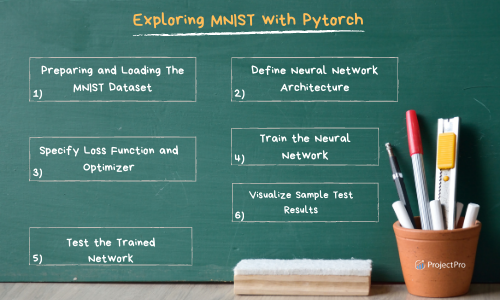100+ Machine Learning Datasets Curated Specially For You

## MNIST Dataset Download - Steps to Follow

Let’s get our hands dirty! While MNIST is also available in the CSV format, for the purpose of this notebook we'll use the original MNIST in ubyte.

Follow these simple steps to download and store MNIST on your local machine:

1. Go to http://yann.lecun.com/exdb/mnist/ and download all the four files(`train-images-idx3-ubyte.gz`, `train-labels-idx1-ubyte.gz`, `t10k-labels-idx1-ubyte.gz`, `t10k-images-idx3-ubyte.gz`) for train images and labels along with test images and labels.
2. In the same directory as your notebook create a folder name `DATA` and inside it creates a folder called `MNIST` and place all these 4 files there.
3. Now, leave it to Pytorch to load the files.

Download the iPython Notebook for end-to-end implementation of Multilayer Perceptron on MNIST Dataset

There are a lot of Deep Learning Frameworks out there that you can use like Keras, Mxnet, Pytorch.

• Keras is an open-source framework for building Artificial Neural Networks and it runs on top of TensorFlow (which provides a low-level implementation of NN), thus providing a layer of abstraction and making it easy to use.
• Mxnet is also another open-source framework provided by Apache. The main advantage of Mxnet is that it’s scalable and supports multiple programming languages.
• We'll be using Pytorch because the code is more Python-like 🐍 and the implementation of the Neural Network is not hidden behind layers of abstraction. So basically, coding ends up being more intuitive.

Click here to view a list of 50+ solved, end-to-end Big Data and Machine Learning Project Solutions (reusable code + videos)

### Import Libraries

You can install torch and torchvison from pytorch.org, choose the applicable OS, language, etc.

Okay, time to load some libraries we will be needing.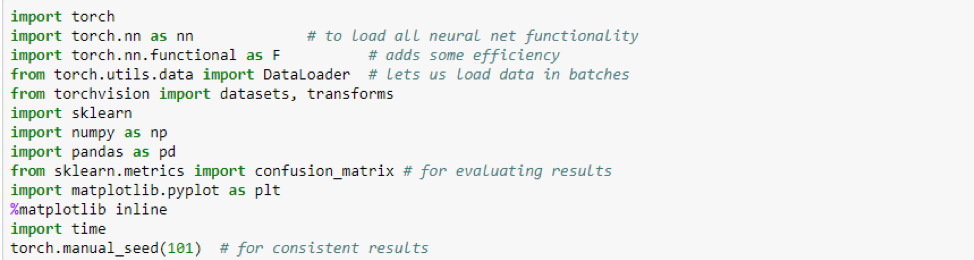### Data Preparation MNIST Dataset

Pytorch has a very convenient way to load the MNIST data using datasets.MNIST instead of data structures such as NumPy arrays and lists. Deep learning models use a very similar DS called a Tensor. When compared to arrays tensors are more computationally efficient and can run on GPUs too. We will convert our MNIST images into tensors when loading them. There are lots of other transformations that you can do using torchvision.transforms like Reshaping, normalizing, etc. on your images but we won't need that since MNIST is a very primitive dataset.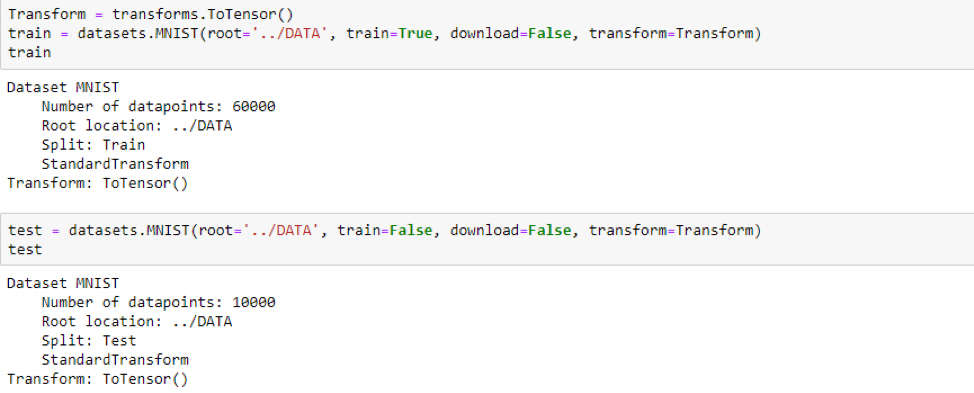Free access to solved code Python and R examples can be found here (these are ready-to-use for your Data Science and ML projects)

### Visualizing a Batch of Training Data from the MNIST Dataset

The train data has 60,000 images and the test has 10,000. Let's look at one.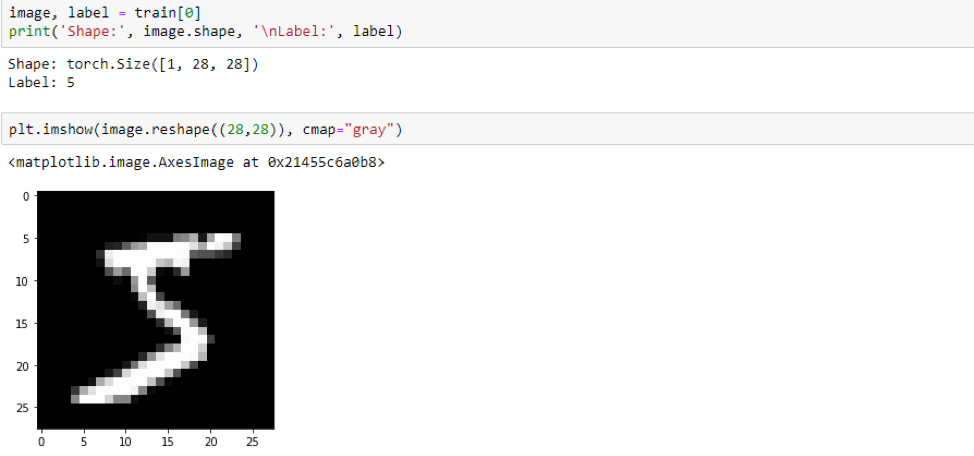Each image is made up of 28X28 pixels. The 1 in torch.size stands for the number of channels, since it's a grayscale image there's only one channel.

Before we go any further, the neural network we will be using is the most basic one. So, let’s have a quick introduction.

## Multilayer Perceptron on MNIST Dataset

• A multilayer perceptron has several Dense layers of neurons in it, hence the name multi-layer.

• These artificial neurons/perceptrons are the fundamental unit in a neural network, quite analogous to the biological neurons in the human brain. The computation happening in a single neuron can be denoted by the equation. N = Wx + b, where x denotes the input to that neuron and W,b stands for weight and bias respectively. These two values are set at random initially and then keep on updating as the network learns.
• Each neuron in a layer is connected to every other neuron in its next layer. In MLPs, data only flows forwards hence they are also sometimes called Feed-Forward Networks.

There are 3 basic components:

1. Input Layer- The input layer would take in the input signal to be processed. In our case, it's a tensor of image pixels.

2. Output Layer- The output layer does the required task of classification/regression. In our case, it outputs one of the 10 classes for digits 0-9 for a given input image.

3. Hidden Layers - There is an arbitrary number of hidden layers in between the input and output layer that do all the computations in a Multilayer Perceptron. The number of hidden layers and the number of neurons can be decided to keep in mind the fact that one layer's output is the next layer's input.

Now, we know the basics of architecture. To understand the working better let's take the example of our use case- image classification with MNIST.

Download the iPython Notebook for end-to-end implementation of Multilayer Perceptron on MNIST Dataset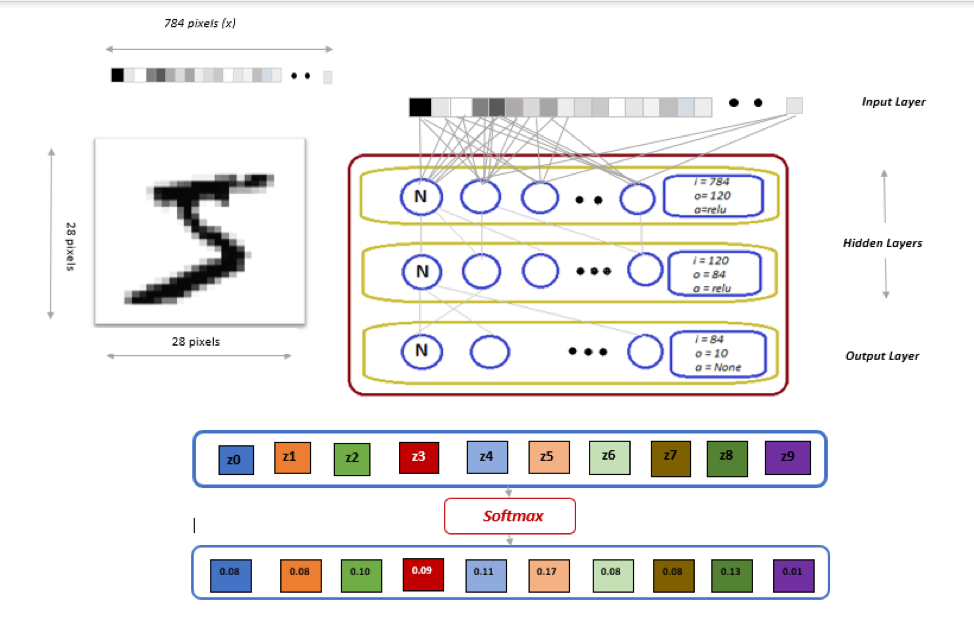I'll try to break down the process into different steps:

1. The pixels in the 28X28 handwritten digit image are flattened to form an array of 784-pixel values. Nothing heavy going on here, just decompressing a 2D array into one dimension.
2. The function of the input layer is just to pass-on the input (array of 784 pixels) into the first hidden layer.
3. The first hidden layer is where the computations start. It has 120 neurons that are each fed the input array. After calculating the result from the formula stated above, each neuron generates an output that is fed into each neuron of the next layer. Except, there is a little twist here. Instead of just simply passing on the result of Wx+b, an activation is calculated on this result.

The activation function is used to clip the output in a definite range like 0-1 or -1 to 1, these ranges can be achieved by Sigmoid and Tanh respectively. The activation function we have used here is ReLu. The main advantage of using the ReLu function is that it does not activate all the neurons at the same time thus making it more computationally efficient than Tanh or Sigmoid.In short, ReLu clips all the negative values and keeps the positive values just the same.

1. The same thing happens in the second hidden layer. It has 84 neurons and takes 120 inputs from the previous layer. The output of this layer is fed into the last layer which is the Output Layer.
2. The Output Layer has only 10 neurons for the 10 classes that we have(digits between 0-9). There isn't any activation function in the output layer because we'll apply another function later.
3. The Softmax takes the output of the last layer(called logits) which could be any 10 real values and converts it into another 10 real values that sum to 1. Softmax transforms the values between 0 and 1, such that they can be interpreted as probabilities. The maximum value pertains to the class predicted by the classifier. In our case, the value is 0.17 and the class is 5.The process described above is a single forward pass through the network and instead of just sending one image as input in a pass, a batch of images is fed in a single pass.

But how does the network learn?

After a single pass through the network, the prediction of the model for that batch of images is compared with the actual labels of those images, and a loss is calculated. Based on the value of this loss, a gradient flow backward through the neural network to update weights(W and b) in each layer. This process is called Backpropagation.

In the next iteration, the neural network would do a slightly better job while predicting. This process of forward-pass and backpropagation keeps on repeating as we try to minimize our loss and we the end of our training.

Now, that we know most of the things, let's dive right into the code.

Loading data into batches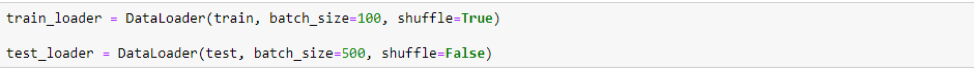• From the 60,000 training records, our images would be sent in batches of 100 through 600 iterations.
• For training, setting a smaller batch size will enable the model to update the weights more often and learn better, but there's a caveat here with smaller batch sizes. This is a hyperparameter that could be tuned, I would suggest you try smaller and larger batch sizes than 100 and see the results.
• During testing, no learning or flow of gradients takes place. So, you can keep the batch size as big as can fit in your RAM.
• Setting shuffle to True means that the dataset will be shuffled after each epoch.

###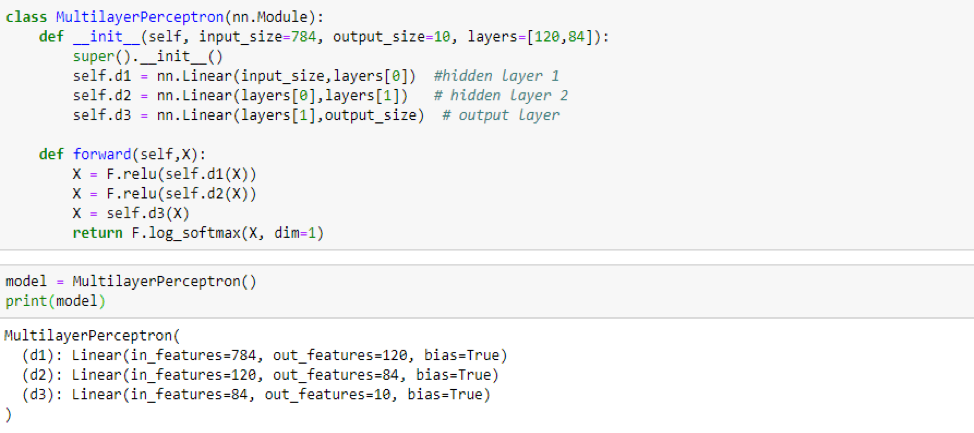• The code is straightforward. In Pytorch there isn't any implementation for the input layer, the input is passed directly into the first hidden layer. However, you'll find the InputLayer in the Keras implementation.
• The number of neurons in the hidden layers and the number of hidden layers is a parameter that can be played with, to get a better result.

### Specify the Loss Function and the Optimizer

Defining loss function and the optimizer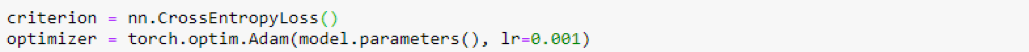There are a lot of loss functions out there like Binary Cross Entropy, Mean Squared Error, Hinged loss etc. The choice of the loss function depends on the problem at hand and the number of classes. Since we are dealing with a Multi-class classification problem, Pytorch's CrossEntropyLoss is our go-to loss function.

Let us talk about the elephant in the room -- the optimizer. Remember, I mentioned that during Backpropagation, we update the weights according to the loss throughout the iterations. We basically try to minimize loss as we move ahead through our training. This process is called optimization. Optimizers are algorithms that try to find the optimal way to minimize the loss by navigating the surface of our loss function. We use Adam because it's the best optimizer out there, as proven by different experiments in the scientific community.

### Train the Neural Network- It's time to train our image classification model!

Before I write a plethora of code for training, let me explain a few concepts that'll be used.

• Epoch - An epoch is a single pass through our full training data(60,000 images). An epoch consists of training steps, which is nothing, but the number of batches passed to the model until all the training data is covered.

It could be expressed as number of training steps = number of training records/batch size, which is 600(60000/100) in our case. We'll train the model for 10 epochs- the model will see the full training data exactly 10 times.

• Flattening the image - Instead of sending the image as a 2D tensor, we flatten it in one-dimension.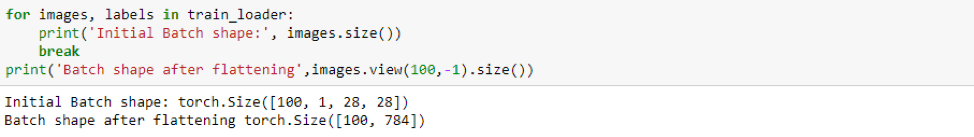The code for training is a few-lines in Keras. As you can see, in Pytorch it's way more because there are wrappers only for very essential stuff and the rest is left to the user to play with. In Pytorch, the user gets a better control over training and it also clears the fundamentals behind model training which is necessary for beginners.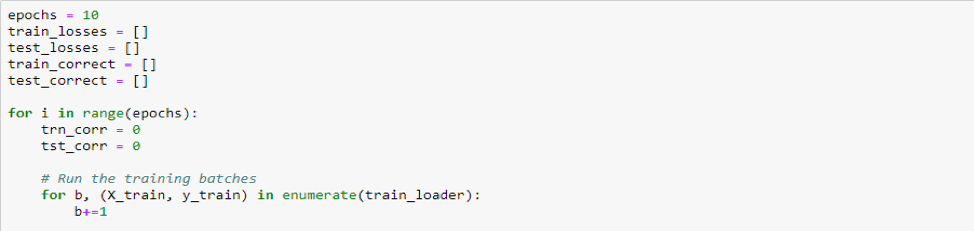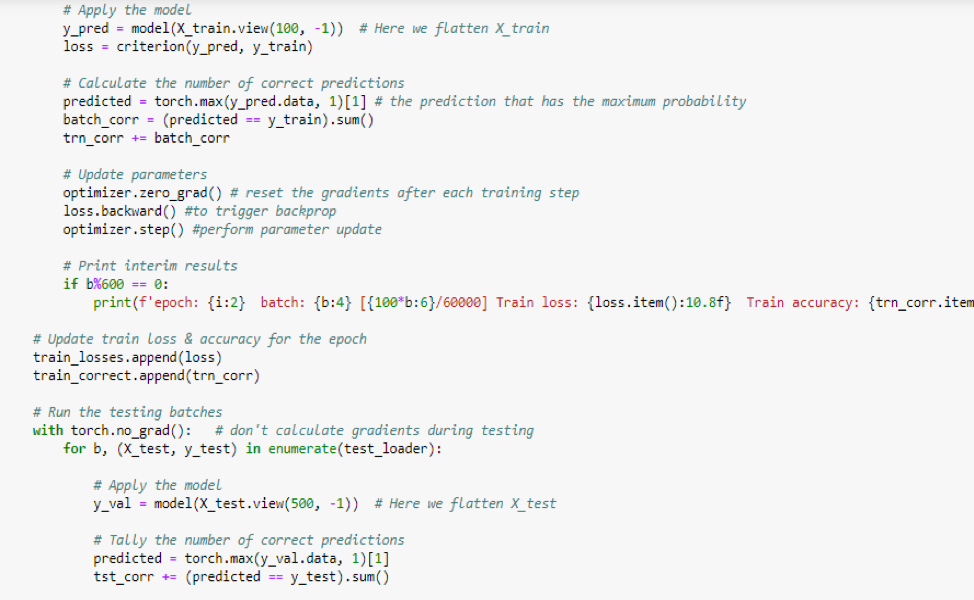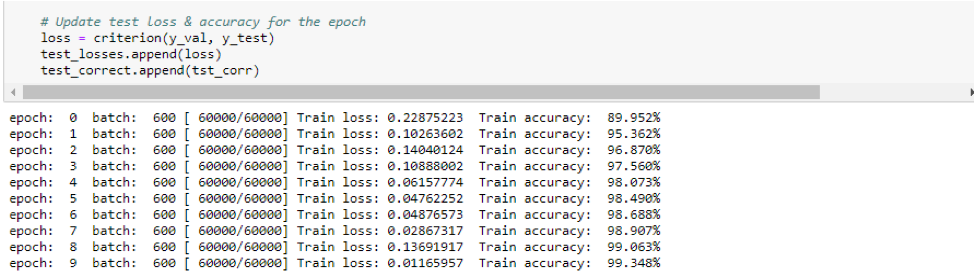### Test the Trained Neural Network

The training loss keeps on decreasing throughout the epochs and we can conclude that our model is definitely learning. But to gauge the performance of our model we'll have to see how well it does on unseen(test) data.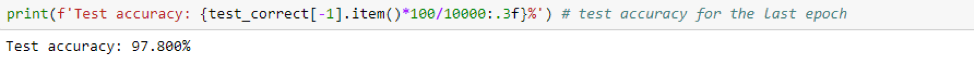Predictions are made on our test data after training completes in every epoch. Since our model continually keeps getting better, the test accuracy of the last epoch is the best.

### Visualizing the Test Results

It will be intuitive and fun to see the progression of loss and accuracy through the epochs.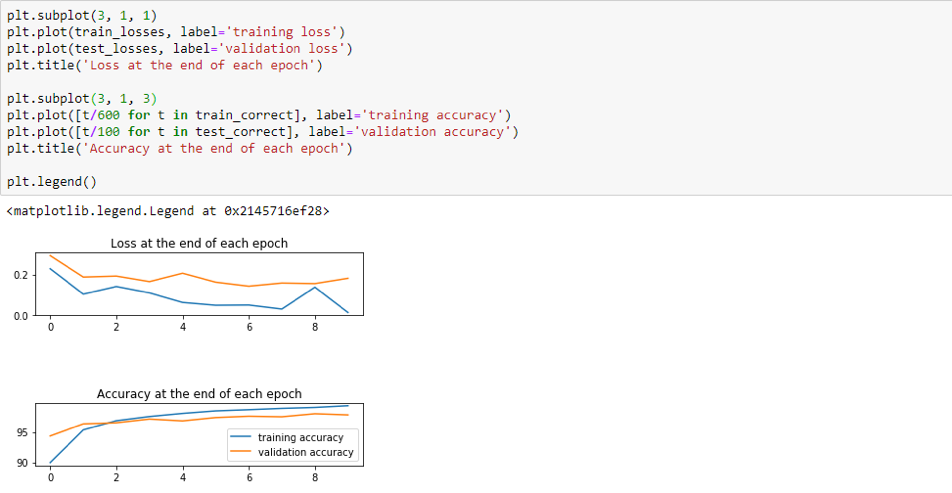### Ending Notes

We are at the end and have successfully trained an image recognition model on MNIST dataset.

There are several tricks you can try to improve the performance of the model like:

• Changing the learning rate in the optimizer.
• Decreasing/Increasing the batch size for training.
• Changing the number of Neurons in the hidden layers.
• Changing the number of hidden layers, while remembering that a layer’s output is the subsequent layer’s input.
• You can also try using another optimizer instead of Adam like RMSProp, Adagrad, etc.

Handwriting recognition from images isn't only limited to MNIST or understanding the basics of Deep Learning - there is a whole field based around it called OCR or Optical Character Recognition. OCR is very useful in digitalizing handwritten documents and is also used by Google Lens to extract text from images.

 PREVIOUS NEXT